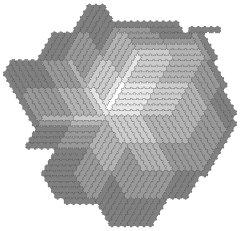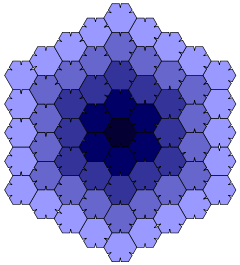# Heesch's problemA polygon with Heesch number 5, the highest finite such number known, found by Casey Mann

In geometry, the Heesch number of a shape is the maximum number of layers of copies of the same shape that can surround it. Heesch's problem is the problem of determining the set of numbers that can be Heesch numbers. Both are named for geometer Heinrich Heesch, who found a tile with Heesch number 1 (the union of a square, equilateral triangle, and 30-60-90 right triangle) and proposed the more general problem.

For example, a square may be surrounded by infinitely many layers of congruent squares in the square tiling, while a circle cannot be surrounded by even a single layer of congruent circles without leaving some gaps. The Heesch number of the square is infinite and the Heesch number of the circle is zero. In more complicated examples, such as the one shown in the illustration, a polygonal tile can be surrounded by several layers, but not by infinitely many; the maximum number of layers is the tile's Heesch number.

## Formal definitions

A tessellation of the plane is a partition of the plane into smaller regions called tiles. The zeroth corona of a tile is defined as the tile itself, and for k > 0 the kth corona is the set of tiles sharing a boundary point with the (k  1)th corona. The Heesch number of a figure S is the maximum value k such that there exists a tiling of the plane, and tile t within that tiling, for which that all tiles in the zeroth through kth coronas of t are congruent to S. In some work on this problem this definition is modified to additionally require that the union of the zeroth through kth coronas of t is a simply connected region.

If there is no upper bound on the number of layers by which a tile may be surrounded, its Heesch number is said to be infinite. In this case, an argument based on König's lemma can be used to show that there exists a tessellation of the whole plane by congruent copies of the tile.Ammann's example of a polygon with Heesch number 4

## Example

Consider the non-convex polygon P shown in the figure to the right, which is formed from a regular hexagon by adding projections on two of its sides and matching indentations on three sides. The figure shows a tessellation consisting of 61 copies of P, one large infinite region, and four small diamond-shaped polygons within the fourth layer. The first through fourth coronas of the central polygon consist entirely of congruent copies of P, so its Heesch number is at least four. One cannot rearrange the copies of the polygon in this figure to avoid creating the small diamond-shaped polygons, because the 61 copies of P have too many indentations relative to the number of projections that could fill them. By formalizing this argument, one can prove that the Heesch number of P is exactly four. According to the modified definition that requires that coronas be simply connected, the Heesch number is three. This example was discovered by Robert Ammann.

## Known results

It is unknown whether all positive integers can be Heesch numbers. The first examples of polygons with Heesch number 2 were provided by Fontaine (1991), who showed that infinitely many polyominoes have this property. Casey Mann has constructed a family of tiles, each with the Heesch number 5, which is the highest known. Mann's tiles have Heesch number 5 even with the restricted definition in which each corona must be simply connected.

For the corresponding problem in the hyperbolic plane, the Heesch number may be arbitrarily large.

## References

1. Mann, Casey (2004), "Heesch's tiling problem", American Mathematical Monthly, 111 (6): 509–517, doi:10.2307/4145069, MR 2076583.
2. Heesch, H. (1968), Regülares Parkettierungsproblem, Cologne and Opladen: Westdeutscher Verlag. As cited by Grünbaum & Shephard (1987) and Fontaine (1991).
3. Dutch, Steven. "The Heesch Tile: An Interesting Non-Tiler". Natural and Applied Sciences, University of Wisconsin–Green Bay. Retrieved 2008-12-22.
4. Grünbaum, Branko; Shephard, G. C. (1987), "Heesch's Problem", Tilings and Patterns, W. H. Freeman, pp. 155–156.
5. Grünbaum & Shephard (1987), 3.8.1 The Extension Theorem, p. 151.
6. Fontaine, Anne (1991), "An infinite number of plane figures with Heesch number two", Journal of Combinatorial Theory, Series A, 57 (1): 151–156, doi:10.1016/0097-3165(91)90013-7.
7. Тарасов, А. С. (2010), О числе Хееша для плоскости Лобачевского [On the Heesch number for the hyperbolic plane], Matematicheskie Zametki (in Russian), 88 (1): 97–104, doi:10.4213/mzm5251, MR 2882166. English translation in Math. Notes 88 (1–2): 97–102, 2010, doi:10.1134/S0001434610070096.